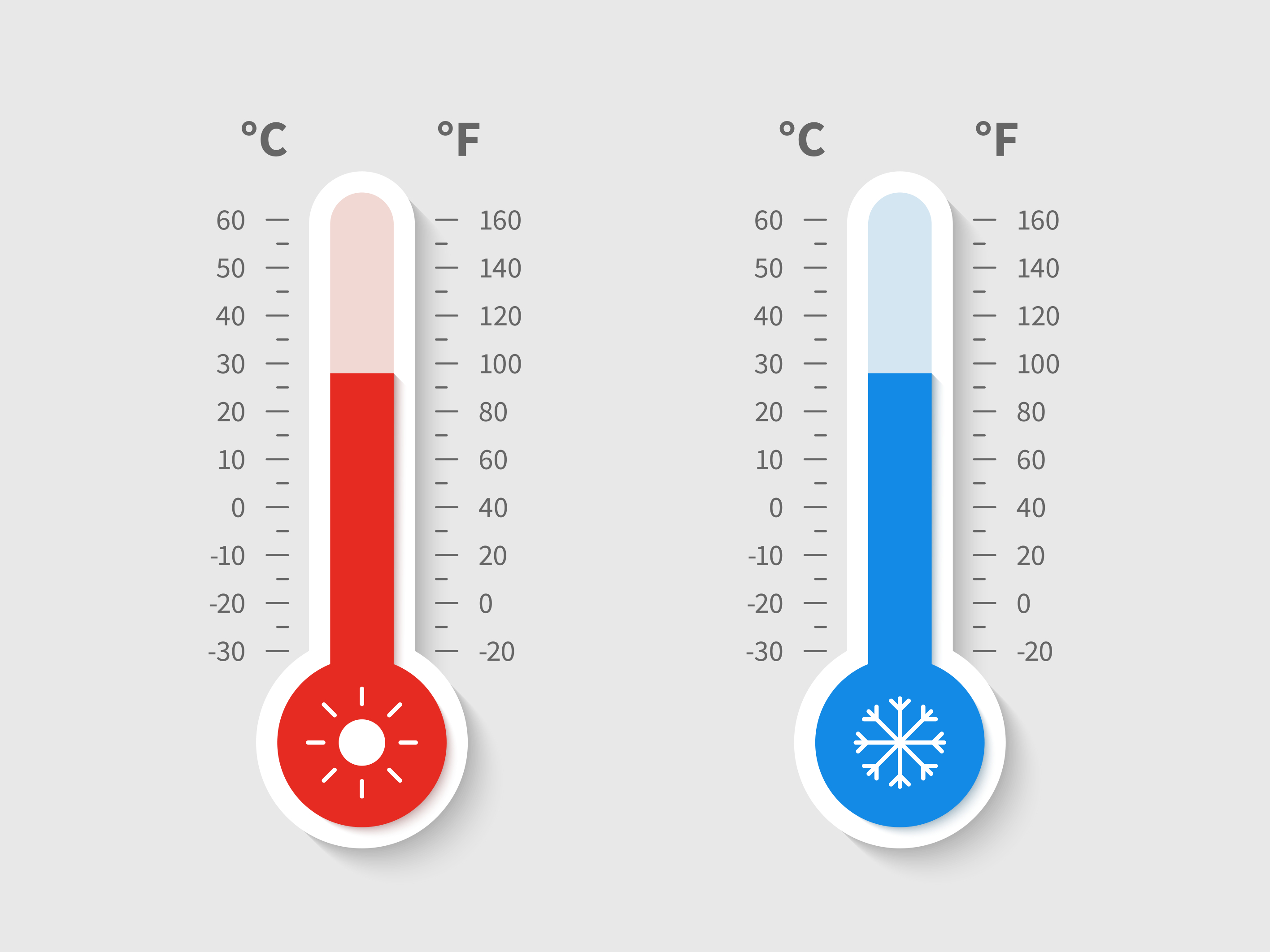×# Introduction

The term "absolute temperature" refers to a measurement of heat that is not relative to anything else. Kelvin (K) is the SI unit for temperature, and 1 K is equal to -273.15 degrees Celsius (°C). Thermodynamic principles, namely the ideal gas law, provide the foundation for the absolute temperature scale. In thermodynamics, absolute temperature plays a crucial role since it is utilized to determine a system's energy. Higher absolute temperatures also mean that a system has more energy. Thus, thermodynamic temperature is another name for absolute temperature.

# What is absolute zero temperature?

Absolute zero is the lowest temperature that could ever exist on Earth, and it's the simplest definition there is. Conventionally, the Kelvin temperature scale is used for this purpose. This scale begins at 0, representing absolute zero. Converting it to degrees Celsius or Fahrenheit may help explain things further. At the absolute temperature, any kind of heat is also impossible.

At this temperature, all of the minuscule particles inside a perfect crystal will stay put and the entropy reaches zero. The concept seems far-fetched, and many people have trouble accepting it as true because of the lack of proof it provides.

It was assumed that articles lose all of their energy and cease to move when the temperature drops to absolute zero. However, according to quantum physics, particles retain some energy even after having all of their other energy eliminated, a phenomenon known as zero point energy. Heisenberg's uncertainty principle asserts that the more information about a particle's location, the less information may be known about its momentum, and vice versa. Particles can't be made stationary entirely since doing so would reveal their precise location and velocity.

Theorists in the field of thermodynamics have been striving for a long time to achieve a temperature of zero. Thus yet, the findings have not proven definitive. Today's sub-zero conditions are the consequence of the application's use of cryocoolers and dilution refrigerators.

People have managed to achieve temperatures very near to absolute zero; the highest yet recorded was 100 pK above absolute zero. Any attempt to chill an object to near absolute zero is problematic since any contact with the object would cause it to warm again. When it comes to getting things down to extremely low temperatures, scientists often turn on lasers to slow down the atoms.Temperature scale

# How is absolute temperature measured?

As the name implies, absolute temperature is the temperature measured on the Kelvin scale, where absolute zero is the absolute minimum. At absolute zero, the velocity of individual particles in matter is at its slowest (minimum energy). As the thermodynamic temperature is considered "absolute," the degree symbol is not appended to it. That is 273 kelvin, not 273 degrees celsius.

The Celsius scale may have its roots in the Kelvin scale, but its units are not absolute, hence it cannot be used to determine absolute temperature. Another absolute temperature scale is the Rankine scale, which uses a degree interval with the Fahrenheit system. Neither Celsius nor Fahrenheit is an absolute temperature scale.

# Temperature Scales

In the present day, three primary temperature scales are in widespread use.

• Kelvin scales are the most widely used, which is used to measure the coldest temperatures possible, zero represents the coldest possible temperature.

• Just like the Kelvin scale, the Rankine zero corresponds to absolute zero. One degree Fahrenheit is equivalent to one Rankine.

• The value of a measurement is inverted on the Delisle scale. Temperatures above freezing are warmer than those below, and vice versa.

# Absolute temperature in terms of Kinetic energy

For an ideal gas, the average kinetic energy (K) of a molecule, at temperature T,  is given as:

K= (3/2) kBT

Where, kB is Boltzmann constant.

If T=0 then K=0.

Thus the molecule will possess no kinetic energy at absolute zero.

Since the value of kinetic energy can never be negative, that value of temperature has to be the lowest possible value.

# Conclusion

The concept of absolute temperature refers to a temperature measurement that is not relative to any particular scale. On the thermodynamic temperature scale, it is denoted with the letter K, which stands for the unit of measurement known as the kelvin (K). The temperature of a system as measured from absolute zero, which is the lowest temperature that can be theoretically conceived of, is referred to as the absolute temperature. In thermodynamics, absolute temperature is used to determine how much energy a system has. In quantum mechanics, absolute temperature is used to determine how much energy individual particles have. The idea of absolute temperature is an essential one in the field of physics, and it is put to use to describe how different temperatures affect the behavior of matter.

# 1. What is the relationship between absolute temperature and energy?

The relationship between absolute temperature and energy is that as temperature increases, so does the average kinetic energy of the particles in a system.

# 2.What is the difference between absolute temperature and relative temperature?

The difference between absolute temperature and relative temperature is that absolute temperature is measured on an absolute temperature scale, such as Kelvin, while relative temperature is measured on a relative temperature scale, such as Celsius.

# 3. What is the relationship between absolute temperature and entropy?

The relationship between absolute temperature and entropy is that as temperature increases, so does the entropy of a system. This is because, at higher temperatures, the molecules of the system have more energy and are able to move around more freely, resulting in a higher entropy.

#### Recent Posts